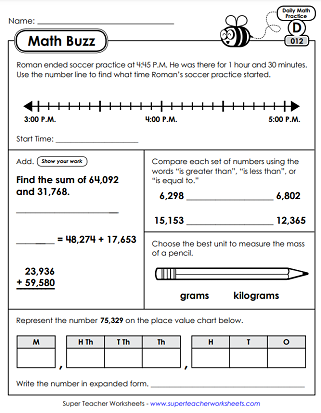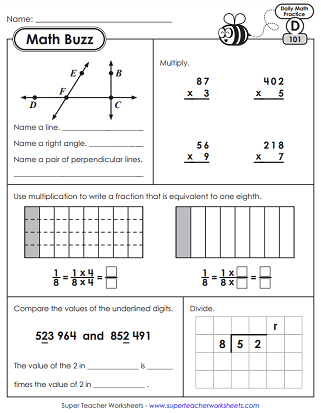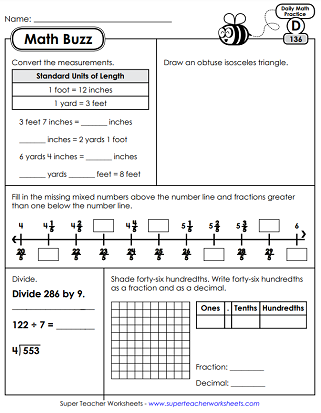# Daily Math Review Worksheets - Level D (4th Grade)

With the help of these daily math practice worksheets, your students will be able to review essential math skills throughout the school year. Each one features common core-aligned, spiraling math problems to solve.This is the first week of the 4th grade math buzz series. This file contains 5 worksheets, reviewing basic skills from the previous grade. Skills covered include: place value, linear measurement, multiplication facts, and comparing fractions.
Review word problems, area, rounding, basic division, elapsed time, comparing fractions, expanded form, and measurement to the nearest quarter inch.
The third week of Math Buzz for 4th grade features skills like: division, place value, advanced subtraction, rounding, fractions, area, and perimeter.
In week 4 of Math Buzz, students will practice comparing numbers, place value, rounding, perimeter, capacity, and division.
Within this set you'll find a variety of word problems, geometry, place value up to 7 places, fractions, advanced addition and subtraction.
Students will solve word problems, find perimeter, standard and expanded form, geometry, fractions on number lines, comparing numbers, and more.
In the seventh set, students will solve input and output tables, practice rounding, geometry, comparing numbers, perimeter, and much more.
In this file for Math Buzz, Week 8, your students will review standard form and expanded form, place value, input and output tables, area, geometry, and rounding.
In week 9 of Math Buzz, have your class practice place value, comparing numbers, division, fractions, patterns, word problems, and input/output tables.
Review order of operations, factoring, word problems, parallel/intersecting/perpendicular lines, adding fractions, and rounding.
More review of 2-digit by 1-digit multiplication, multi-step story problems, acute/right/obtuse angles, subtraction across zero, and liter/milliliter conversions.
In this set, students will analyze multi-digit multiplication patterns, solve area patterns, answer a capacity story challenge, and fill in multiples of twelve.
In this set, we have more word problems, symmetry challenges, comparing fractions, part-part-whole models, acute/right/obtuse angle identification, factor pairs, and a line plot.
Multiply 3-digit by 1-digit numbers, add fractions with like denominators, draw line segments, and solve an area word problem.
Multiply a fraction by a whole number, use illustrations to practice division with remainders, review place value, find an equivalent fraction, and review area & perimeter.
Write fractions on a number line. Multiply whole numbers by fractions. Decompose a rectangle to find equivalent fractions. Find quotients with remainders.
This file contains the next 5 days of 4th grade math review. Problems assess the following skills: number patterns, fractions on a number line, 3-digit by 1-digit multiplication, prime/composite numbers, inches to feet conversions, and more.
On these worksheets, students will practice the following skills: classify angles (acute/obtuse/right), lines of symmetry, multiplying multi-digit numbers by a single digit, and division word problems.
Review number patterns, pounds to ounces conversions, angle types, fractions on a number line, division with remainders, and equivalent fractions.
Answer questions related to right angles, perpendicular lines, value of underlined digits, ordering fractions, multi-step word problems, and types of angles.
Challenge your 4th graders to solve math problems related to: multi-step word problems, decomposing fractions, prime and composite numbers, seconds to minutes conversions, and geometry.
Read & interpret data on a line plot. Use a model to solve 2-digit by 2-digit multiplication problems. Convert miles to feet and days to weeks. Add fractions with like denominators.
Practice multi-digit multiplication, number patterns, triangle classifications, measuring angles with a protractor, and line plots.
Use fraction models to write equations, complete a long division problem, measure with a protractor, convert from meters to kilometers, calculate elapsed time on a number line, and create a line plot.
This set begins with a place value word problem. There are also questions related to operations with fractions, converting and comparing feet to inches, finding equivalent fractions, area models, and division with 3-digit dividends.
Learn about decimal place value, as well as decimal-fraction relationships. Solve long division problems with 3-digit dividends and 1-digit divisors. Shade models to represent mixed numbers. Add angles. Multiply 2-digit by 2-digit numbers using the standard algorithm.
Convert between feet, yards, and inches. Write improper fractions and mixed numbers on a number line. Review decimals and fractions. Solve a word problem related to elapsed time and angles. Find area and perimeter. Determine the missing addend in fraction addition problems.
Add and subtract mixed numbers. Answer questions related to area and perimeter. Name the polygons. Multiply whole numbers by fractions. Compare decimals.
This is the final week of 4th grade Math Buzz worksheets. If you've made it this far - congratulations! You've reached the end. In this set, students answer questions related to operations with fractions and mixed numbers, classifying quadrilaterals, multiplying fractions by whole numbers, and word problems with decimal operations.
More Daily Math Review (Math Buzz)

We have Math Buzz, Daily Math Review, for all grade levels.

This page has a collection daily word problems that you can practice with your 4th graders. Includes single and multiple-step problems covering a wide-range of skills.

## Sample Worksheet ImagesMy Account
Site Information